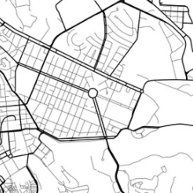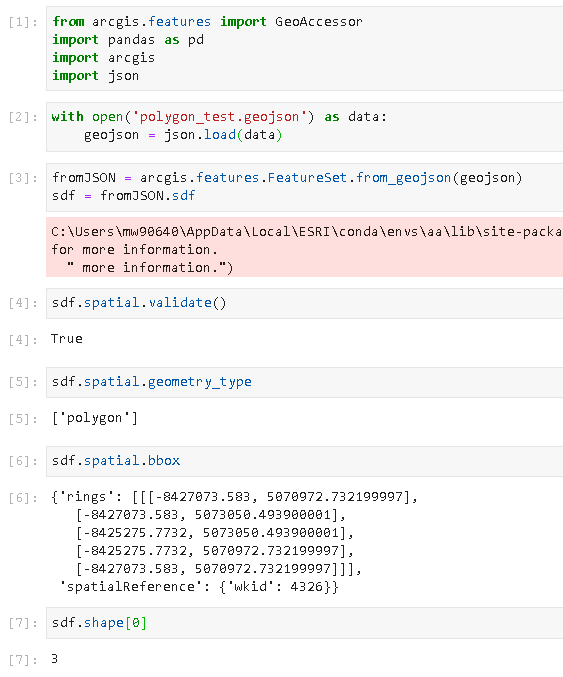# ArcGIS Python API Set Geometry Column of Spatially Enabled Data Frame

4246
7
10-26-2018 09:10 AMNew Contributor III

I am trying to

1. connect to a PostGIS database

2. Create a Spatially Enabled Data Frame

3. Export out to a feature layer

4. Push to AGOL.

Of those steps, I have succeeded at number one, but I am getting a error in the process of setting a geometry column in the DataFrame.

I have the function `set_geomerty()` does not like the format of the data that i am putting in, which has been text and shapely format.  Is there an ESRI specific format that i should be using or a function that can put the geometry into that format?

IDE: Jupyter Notebook

python: 3.6

ArcGIS Python Api: 1.5.0

import pandas as pd

conn = config.conn
df = pd.read_sql("select id, ST_AsText(geom) AS geometry from table where group_id = 63 limit 1", con=conn)
sdf = df.set_geometry('geometry')

bmp_id geometry
0 Lot_5_78 POINT (-121.799248349 36.6987788232)

~/.virtualenv/esri/lib/python3.6/site-packages/arcgis/features/_data/geodataset/geodataframe.py in set_geometry(self, col, drop, inplace, sr)
1799 # Check that we are using a listlike of geometries
1800 if not all(isinstance(item, GEOM_TYPES) or not item for item in level):
-> 1801 raise TypeError("Input geometry column must contain valid geometry objects.")
1802 #if isinstance(frame[geo_column_name], pd.Series):
1803 # frame[geo_column_name] = GeoSeries(frame[geo_column_name])

TypeError: Input geometry column must contain valid geometry objects.

Gracias

7 RepliesNew Contributor III

Update:  I have been able to find a work around by following the post by Joel McCune here , by first converting the PostGIS text as shapely, then converting the shapely geometry as ArcGIS geometry format, which is pretty hacky.  I am sure there is a function that convert PostGIS text / json outputs into ArcGIS geometry.  Any ideas?

Also weird, after running the conversion the correct geometry format, I can run set_geometry on the geom column, publish a feature_layer to AGOL, but I cannot plot on a map within Jupyter Notebook.  I get the

`Exception: Spatial column not defined, please use `set_geometry``

Even though I have run the method 2 lines above.  ¯\_(ツ)_/¯

Full code here:

from arcgis.geometry import Geometry
from arcgis.geometry import BaseGeometry

@classmethod
def from_shapely(cls, shapely_geometry):
return cls(shapely_geometry.__geo_interface__)

BaseGeometry.from_shapely = from_shapely

conn = config.conn
df = pd.read_sql("select id, ST_AsText(geom) AS geometry from ch01.sweet_table where group_id = 1", con=conn)

df['geometry'] = df['geometry'].apply(lambda x: Geometry.from_shapely(x))
Geometry(df.iloc.geometry).is_valid
sdf = df.set_geometry('geometry')

Geometry(sdf.iloc.geometry).is_valid #test geometry is valid

sdf.spatial.plot(map_widget=m) #plotting error

new_lyr_item = sdf.spatial.to_featurelayer('Tester Pester')
published_item = new_lyr_item.publish()New Contributor III

import pandas as pd
from arcgis.features import GeoAccessor, GeoSeriesAccessor

conn = config.conn
sql = “””
SELECT
id,
ST_X(geom) AS X,
ST_Y(geom) AS Y
FROM

ch01.sweet_table
WHERE
group_id = 1”””
sdf = pd.DataFrame.spatial.from_xy(df, 'X', 'Y', sr=4326)

At this point you should have a functioning spatial dataframe without usingNew Contributor III

Hi Per,

Yes, that would work if I were using points, which I know is what I used in the example.  However, the meat and potatoes of the data that i am dealing with is polygon.

I basically need something like the from_xy function for polygons, multipolygons and lines as well.New Contributor III

It’s definitely easier with points- even using polygons imported from a featureclass is painfully slow if the data is large.  What if you convert your data to geojson using something like ogr2ogr?  Then something like this will work:New Contributor III

Yeah, I this is the best solution.  It is unfortunate that there does not seem to be a solution where geometries can be created in ESRI format using the spatial data frame only, but this is a fast solution, once you have the geojson.

Thanks,

NatNew Contributor III

After trying the geojson solution, I am falling back to using shapely and the from_shapely custom method which I took from Joel McCune.  The geojson route ended up also being slow and having lots of geometry errors anyway, so I would need to do some geometry updates either way, so sticking with the "hack".  I have posted an idea in ESRI Ideas to encourage a loads method similar to the one I am using from shapely. Thanks to Per for above recommendations.New Contributor III

Hello!

Have you find the solution? I already have a geodataframe created from postgis and I'm tryng to convert from geodataframe to ESRI spatial enabled dataframe, but I'm not able to.
Thanks!